# Fibonacci Numbers and Nature - Part 2 Why is the Golden section the "best" arrangement?

The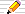icon means there is a Things to do section of questions to start your own investigations.

On the first page on the Fibonacci Numbers and Nature we saw that the Fibonacci numbers appeared in (idealised) rabbit, cow and bee populations, and in the arrangements of petals round a flower, leaves round branches and seeds on seed-heads and pinecones and in everyday fruit and vegetables.
We explained why they appear in the rabbit, cow and bee populations but what about the other appearances that we see around us in nature? The answer relates to why Phi appears so often in plants and the Fibonacci numbers appear because the eye "sees" the Fibonaci numbers in the spirals of seedheads, leaf arrangements and so on, and we looked at this on the previous Fibonacci Numbers in Nature page.

Why does nature like using Phi in so many plants?
The answer lies in packings - the best arrangement of objects to minimise wasted space.

## Packings

If you were asked what was the best way to pack objects your answer would depend on the shape of the objects since....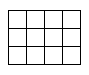...square objects would pack most closely in a square array, whereas round objects pack better in a hexagonal arrangement....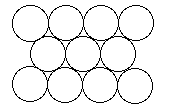So why doesn't nature use one of these? Seeds are round (mostly), so why don't we see hexagonal arrangments on seedheads?
Although hexagonal symmetry IS the best packing for circular seeds, it doesn't answer the question of how leaves should be arranged round a stem or how to pack flower-heads (which are circular because that is the shape that encloses maximum area for minimum edge) with seeds that grow in size.

What nature seems to use is the same pattern to place seeds on a seedhead as it used to arrange petals around the edge of a flower AND to place leaves round a stem. What is more, ALL of these maintain their efficiency as the plant continues to grow and that's a lot to ask of a single process!

So just how do plants grow to maintain this optimality of design?

### The Meristem and Spiral growth patterns

Botanists have shown that plants grow from a single tiny group of cells right at the tip of any growing plant, called the meristem. There is a separate meristem at the end of each branch or twig where new cells are formed. Once formed, they grow in size, but new cells are only formed at such growing points. Cells earlier down the stem expand and so the growing point rises.

Also, these cells grow in a spiral fashion, as if the stem turns by an angle and then a new cell appears, turning again and then another new cell is formed and so on.

These cells may then become a new branch, or perhaps on a flower become petals and stamens.

The amazing thing is that a single fixed angle can produce the optimal design no matter how big the plant grows. So, once an angle is fixed for a leaf, say, that leaf will least obscure the leaves below and be least obscured by any future leaves above it. Similarly, once a seed is positioned on a seedhead, the seed continues out in a straight line pushed out by other new seeds, but retaining the original angle on the seedhead. No matter how large the seedhead, the seeds will always be packed uniformly on the seedhead.

And all this can be done with a single fixed angle of rotation between new cells?
Yes! This was suspected by people as early as the last century. The principle that a single angle produces uniform packings no matter how much growth appears after it was only proved mathematically in 1993 by Douady and Couder, two french mathematicians.

You will have already guessed what the fixed angle of turn is - it is Phi cells per turn or phi turns per new cell.

## Why does the Golden Ratio (Phi) appear in plants?

The arrangements of leaves is the same as for seeds and petals. All are placed at 0·618034.. leaves, (seeds, petals) per turn. In terms of degrees this is 0·618034 of 360° which is 222·492...°.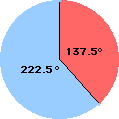However, we tend to "see" the smaller angle which is (1-0·618034)x360 = 0·381966x360 = 137·50776..°. When we look at properties of Phi and phi on a later page, we shall see that
1-phi = phi2 = Phi-2

If there are Phi (1·618...) leaves per turn (or, equivalently, phi=0·618... turns per leaf ), then we have the best packing so that each leaf gets the maximum exposure to light, casting the least shadow on the others. This also gives the best possible area exposed to falling rain so the rain is directed back along the leaf and down the stem to the roots. For flowers or petals, it gives the best possible exposure to insects to attract them for pollination.
The whole of the plant seems to produce its leaves, flowerhead petals and then seeds based upon the golden number.
And why do the Fibonacci numbers appear as leaf arrangements and as the number of spirals on seedheads?

The Fibonacci numbers form the best whole number approximations to the golden number, which we examined in greater detail on the first Fibonacci in Nature page.

Let's now try and show just why phi is the best angle to use in the next few sections of this page.

### Why is the Golden section the "best" number?

The links in this section are to Quicktime animations. They are worth viewing as they show the dynamics of what might happen if seeds were not placed with a phi-angle between them.

Why not 0·6 of a turn per seed or 0·5 or 0·48 or 1·6 or some other number?

First we can agree that turning 0·6 of a turn is exactly the same as turning 1·6 turns or 2·6 turns or even 12·6 turns because the position of the point looks the same. So we can ignore the whole number part of a turn and only examine the fractional part.

Also, since a 0·6 of a turn in one direction is the same as 0·4 of a turn in the other, we could limit our investigation to turns which are less than 0·5 too. However sometimes it will be easier to talk of fractions of a turn which are bigger than 0·5 or even that are bigger than 1, but the only important part of the number is the fractional part.

So, in terms of seeds - which develop into fruit - what is a fruit-ful numbers? Which has the best properties as a turning angle for our meristem? It turns out that numbers which are simple fractions are not good choices, as we see in the next section.

### Why exact fractions are fruitless!

Let's first see what happens with a simple number such as 0·5 turns per seed. Since 0·5=1/2 we get just 2 "arms" and the seeds use the space on the seedhead very inefficiently: the seedhead is long and floppy. The picture is a link to an animation where you can see the new seeds appearing at the centre as the older ones continue growing outwards in a straight line from the central growing point (where the new seed cells appear).

A circular seedhead is more compact and would have better mechanical strength and so be better able to withstand wind and heavy rain.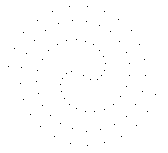Here is 0·48 of a turn between seeds.
[The picture is again a link to an animation.]
The seeds seem to be sprayed from two revolving "arms". This is because 0·48 is very close to 0·5 and a half-turn between seeds would mean that they would just appear on alternate sides, in a straight line. Since 0·48 is a bit less than 0·5, the "arms" seem to rotate backwards a bit each time.
So if we has 0·52 seeds per turn, we would be a little in advance of half a turn and the final pattern would be a mirror-image (as if we had used 1-0·52=0·48 seeds per turn but turning in the opposite direction).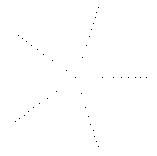What do you think will happen with 0·6 of a turn between successive seeds?
Did you expect it to be so different?
Notice how the seeds are not equally spaced, but fairly soon settle down to 5 "arms". Why?
Because 0·6=3/5 so every 3 turns will have produced exactly 5 seeds and the sixth seed will be at the same angle as the first, the seventh in the same (angular) position as the second and so on. The seeds appearing at every third arm, in turn, round and round the 5 arms. So we count 3-of-the-5 (3/5) to find the next "arm" where a seed will appear.

If we try 1·6 or 2·6 or 3·6 can you see that we will get the same animation since the extra whole turns do not affect where the seeds are placed?

So what seems to be important is just the fractional part of our seeds-per-turn value and we can ignore the whole number part. There is another value that will give the same animation too. What is it? Well, if we went 0·6 of a turn in the other direction, it is equivalent to going 1-0·6=0·4 of a turn between seeds. So also would be 1·4, 1·4, 3·4 and so on.

Here's what happens if we have a value closer to phi(0·6180339..), namely 0·61. You'll notice that it is better, but that there are still large gaps between the seeds nearest the centre, so the space is not best used. This is also equivalent to using 1·61, 2·61, etc. and also to 1-0·61=0·39 and therefore to 1·39 and 2·39 and so on.

In fact, any number which can be written as an exact ratio (a rational number) would not be good as a turn-per-seed angle.
If we use p/q as our angle-turn-between-successive-turns, then we will end up with q straight arms, the seeds being placed every p-th arm. [This explains why 0·6=3/5 has 5 arms and the seeds appear at every third arm, going round and round.]

### The rational answer is an irrational number!

So what is a "good" value? One that is NOT an exact ratio since very large seed heads will eventually end up with seeds in straight lines.
Numbers which cannot be expressed exactly as a ratio are called irrational numbers (ir-ratio-nal) and this description applies to such values as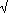2, Phi, phi, e, pi and any multiple of them too.

You'll notice that the e(2·71828...) animation has 7 arms since its turns-per-seed is (two whole turns plus) 0·71828... of a turn, which is a bit more than 5/7(=0·71428..).
A similar thing happens with pi(3·14159..) since the fraction of a turn left over after 3 whole turns is 0·14159 and is close to 1/7=0·142857.. . It is a little less, so the "arms" bend in the opposite direction to that of e's (which were a bit more than 5/7).
These rational numbers are called rational approximations to the real number value.
If we take more and more seeds, the spirals alter and we get better and better approximations to the irrational value.

What is "the best" irrational number?
One that never settles down to a rational approximation for very long. The mathematical theory is called CONTINUED FRACTIONS.
The simplest such number is that which is expressed as P=1+1/(1+1/(1+1/(...) or, its reciprocal p=1/(1+1/(1+1/(...))).
P is just 1+1/P, or P2=P+1.
p is just 1/(1+p) so p2+p=1.
We will see later that these are just definitions of Phi (P) and phi (p) (and their negatives)!
The exact value of Phi is (5 + 1)/2
and of phi is (5 – 1)/2.
Both are irrational numbers whose rational approximations are ...

```  phi:   1/1,  1/2,  2/3,  3/5,  5/8,  8/13,  13/21, ...
Phi:   1/1,  2/1,  3/2,  5/3,  8/5,  13/8,  21/13, ...
```

which is why you see the Fibonacci spirals in the seed heads!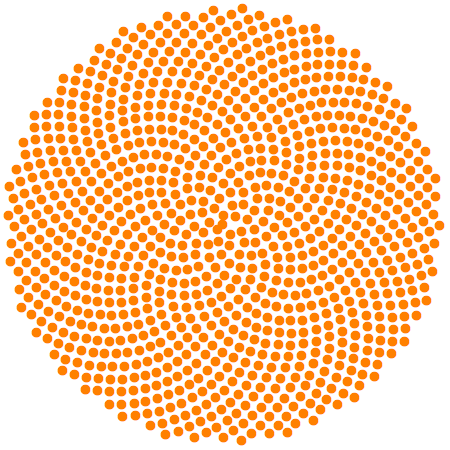1·61803 39887 49894 84820 45868 34365 63811 77203 09179 80576 ..More..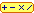## Interactive Demonstrations

### How the demonstrations are made

In all the following interactive demonstrations, the following principles are used in the programming:
1. The seeds are given numbers from 1 up as far as you want to go.

2. The position of a seed s given in polar coordinates which involve two numbers:
• a distance, r, measured from a fixed point called the origin and
• an angle of rotation, theta, measured from a fixed direction called the axis
We use this system in everyday language when we say:
Look out! There's the teacher: 30 metres at 3 o'clock!
where the axis of 12 o'clock is straight ahead.

So we need a centre point or origin and a fixed line through it as an axis to measure the angles from.
A point is then uniquely identified by its distance r from the origin and its angle theta measured as a rotation about the origin from the axis.

1. The distance r of seed number s is √s,
so for example, seed number s = 9 is at a distance 3 from the origin
2. The angle theta that seed s has as seen from the origin is s × phi of a whole turn, where phi = 0.6180339... = (√5 – 1 ) / 2
so for example, seed s = 9 is 9 phi = 9 × 0.61804 = 5.5624 turns.
Since a whole turn takes us back to the starting direction, we can ignore the whole-number part and all we need is the fractional part 0.5624 of a turn but it does no harm to use 5.5624 either.

3.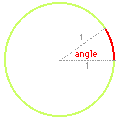To plot the seedhead:
Most computer languages use a graphing system with x and y axes called cartesian coordinates.
They also provide functions on angles where the unit of angle is a radian that is, an angle is measured by the distance it makes around a circle of unit radius.
Because a unit circle has a circumference of 2π, there are 2π = 2 3.14159267... = 6.2831853... radians in one complete turn.
Now we can convert the distance-angle coordinates for a seed into cartesian coordinates as follows:
1. The x-coordinate is r cos( 2 π theta)
2. The y-coordinate is r sin( 2 π theta )
Here is a check on the values for the first 5 seeds:
 s r theta x y 1 1 0.61803399 -0.7373689 -0.6754903 2 1.41421356 1.23606798 0.12363865 1.4087986 3 1.73205081 1.85410197 1.05384702 -1.3745568 4 2 2.47213595 -1.969427 0.3483639 5 2.23606798 3.09016994 1.8866942 1.20016041
Now plot your seeds on an x-y graph using the cartesian coordinates for each seed.
By multiplying the seed number by a different ratio to get its angle, you can experiment and see what seed heads would be like if nature used a different value for its turns-per-seed value too.

Click on the thumbnail images or links to open a demonstration in a new window.

### Quicktime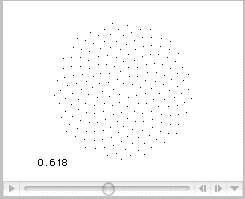Here is another Quicktime movie which shows various turns-per-seed values near phi (0·61803) showing that there are always gaps towards the outer edge of the "seedhead" and that phi gives the best value for all sizes of flowerhead.
Depending on your browser, you should be able to move the slider to any part of the movie to view individual frames and values near phi.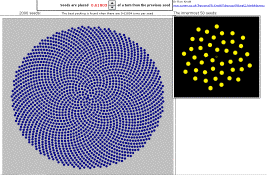This Excel spreadsheet employs a slider to alter the turns-per-seed on an interactive chart to show that 0.61804 is better than 0.61803 or 0.61805 in terms of the evenness of the distribution of 2000 seeds on a seedhead. A smaller view of the innermost 50 seeds is given as well. It does not use Macros so disable them if asked when you load it.

### Mathematica

There are some nice interactive phyllotaxis demonstrations made with Mathematica from Wolfram Research in their Demonstrations Project.
Each can either be viewed without Mathematica in a browser page (as a movie of the demonstration) or by downloading the free Mathematica Player in which case the demonstration is fully interactive.

### Maple

Waterloo Software's Maple is another professional mathematics package similar to Mathematica. The Maple code here illustrates the algorithm used to generate a diagram of seeds with a given number of turns-per-seed.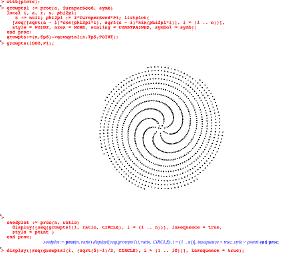The Maple code: > with(plots): > growpts1 := proc(n, TurnperSeed, symb) local i, a, r, s, phi2pi; s := null; phi2pi := 2*TurnperSeed*Pi; listplot( [seq([sqrt(n - i)*cos(phi2pi*i), sqrt(n - i)*sin(phi2pi*i)], i = (1 .. n))], style = POINT, axes = NONE, scaling = CONSTRAINED, symbol = symb); end proc: > growpts:=(n,TpS)->growpts1(n,TpS,POINT): > growpts(1000,Pi); > seedplot := proc(n, ratio) display([seq(growpts1(i, ratio, CIRCLE), i = (1 .. n))], insequence = true, style = point ) end proc; seedplot := proc(n, ratio) display([seq(growpts1(i, ratio, CIRCLE), i = (1 .. n))], insequence = true, style = point) end proc; > display([seq(growpts1(i, (sqrt(5)-1)/2, CIRCLE), i = (1 .. 10))], insequence = true);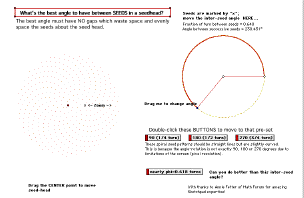Try this Geometer's Sketchpad active demonstration which lets you alter the inter-seed angle at will (and animate it) to see just why the golden section angle produces the best packing.
Geometer's Sketchpad is available as a free trial for PC and Apple Mac for teachers and administrators.

1·61803 39887 49894 84820 45868 34365 63811 77203 09179 80576 ..More..####You do the maths...1. The "rational approximations" to real numbers are better seen if, instead of producing seeds at the centre, we keep adding them round the outside - that is, along the square-root spiral which has equation R=A where R is the (radial) distance of a point from the origin, and A its angle turn (from the 0 angle direction). Use any of the Demonstrations to "grow plants" that will find good rational approximations to a decimal fraction of your choice. For example, Pi as the angle of rotation between seeds, shows 7 arms clearly after only 100 seeds, gets confused at about 500 seeds but by 1000 shows a better approximation - there are 113 "arms", seeds being grown every 16 showing that a better approximation for Pi is 3+16/113=355/113. [As Jordi Mas pointed out to me, this approximation for pi was known in China as far back as the year 500!]
2. What about approximations to sqrt(3) or sqrt(5)?
3. Take sqrt(3) and plot lots of "seeds".
What sequence of approximations do you get? You should be able to answer this if you plot 500 seeds.
4. Now convert each approximation into a continued fraction. What pattern in the numbers in the continued fraction emerges?
5. Try to prove that the pattern continues indefinitely, by proving its value is sqrt(3).

1·61803 39887 49894 84820 45868 34365 63811 77203 09179 80576 ..More..### Phyllotaxis

The technical term for the study of the arrangements of leaves and of seedheads in plants is phyllotaxis.

• An important technical paper about phi and its optimal properties for plant growth can be found in Phyllotaxis as a self-organised growth process by Stephane Douady and Yves Couder, pages 341 to 352 in Growth Patterns in Physical Sciences and Biology, (editor J M Garcia-Ruiz et al), Plenum press, 1993.
• A history of the study of phyllotaxis by I Adler, D Barabe, R V Jean in Annals of Botany, 1997, Vol.80, No.3, pp.231-244.
• A better way to construct the Sunflower head in Mathematical Biosciences volume 44, (1979) pages 145 - 174.

### Fibonacci Numbers in Nature

Here are some not-too technical papers about the maths which justifies the occurrence of the Fibonacci numbers in nature:
• A H Church On the relation of Phyllotaxis to Mechanical Laws, published by Williams and Norgat, London 1904.
• Phyllotaxis, anthotaxis and semataxis Acta Biotheoretica Vol 14, 1961, pages 1-28.
• Phyllotaxis: Its Quantitative Expression and Relation to growth in the Apex Phil. Trans. Series B Vol 235, 1951, pages 509-564.
• D'Arcy W Thompson On Growth and Form Dover Press 1992.
This is the complete edition! (Click on the title-link for more information and to order it now.)
There is also an abridged version from Cambridge University press (more information and order it on line via the title-link.)
• T A Davis, Fibonacci Numbers for Palm Foliar Spirals Acta Botanica Neelandica, Vol 19, 1970, pages 236-243.
• T A Davis Why Fibonacci Sequence for Palm Leaf Spirals?, Fibonacci Quarterly, Vol 9, 1971, pages 237-244.
• The Algorithmic Beauty of Plants by P Prusinkiewicz, and A Lindenmayer, published by Springer-Verlag (Second printing 1996) is an astounding book of wonderful images and patterns in plant shapes as well as algorithms for modelling and simulation by computer. (For more information and how to order it online use the title-link).
Related to this book is:
• The Algorithmic Beauty of Sea Shells (Virtual Laboratory) in hardback by Hans Meinhardt, Przemyslaw Prusinkiewicz, Deborah R. Fowler (more information and order it online via this title-link).
• The Curves of Life: Being an Account of Spiral Formations and Their Application to Growth in Nature, to Science, and to Art Sir Theodore A Cook, Dover books, 1979, ISBN 0 486 23701 X.
A Dover reprint of a classic 1914 book. (More information and you can order it online via the title-link.)
• Also see H S M Coxeter's Introduction to Geometry, published by Wiley, in its Wiley Classics Library series, 1989, ISBN 0471504580, especially chapter 11 on Phyllotaxis. (More information and order it online via the title-link.)

© 1996-2016 Dr Ron Knott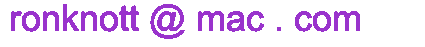updated 23 June 2017